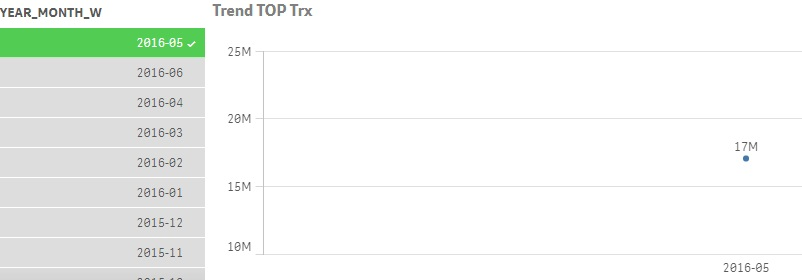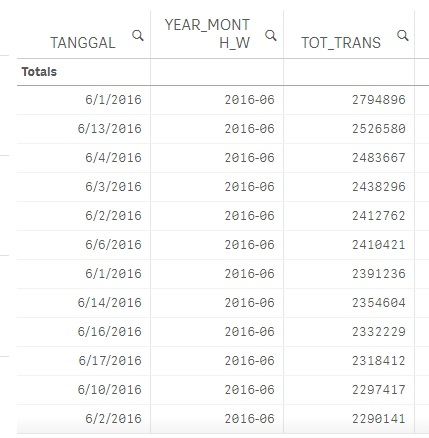# QlikView Scripting

Discussion Board for collaboration on QlikView Scripting.

Announcements

Not applicable

## 12 months Trend for Max Transaction

Hello everybody,

I have a problem, why my 12 months Trend Line only show 1 month value when I select a Year_month in QlikSense?

This is my Measure : Max(Aggr(sum({\$<YEAR_MONTH_W = {">=\$(=date(addmonths(max(YEAR_MONTH_W), -12), 'YYYY-MM'))"} * {"<=\$(=max(YEAR_MONTH_W))"}>}TOT_TRANS),TANGGAL)).

If I don't select any Year_month, the Trend line is OK.Best Regards

Dmw

5 Replies
Not applicable

## Re: 12 months Trend for Max Transaction

You have to bypass YEAR_MONTH filter in your expression...

what is your expression in expression tab?

Not applicable

## Re: 12 months Trend for Max Transaction

=Max(Aggr(sum({\$<YEAR_MONTH_W = {">=\$(=date(addmonths(max(YEAR_MONTH_W), -12), 'YYYY-MM'))"} * {"<=\$(=max(YEAR_MONTH_W))", YEAR_MONTH_W=}>}TOT_TRANS),TANGGAL))

Not applicable

## Re: 12 months Trend for Max Transaction

HI,

I have changed the expression with : Max(Aggr(sum({\$<YEAR_MONTH_W = {">=\$(=date(addmonths(max(YEAR_MONTH_W), -12), 'YYYY-MM'))"} * {"<=\$(=max(YEAR_MONTH_W))", YEAR_MONTH_W}>}TOT_TRANS),TANGGAL))

But the Result is still the same. It only shows 1 month value when I select a Year_month.

Best Regards

Not applicable

## Re: 12 months Trend for Max Transaction

Hi,

Sorry, what do you mean with Expression in expression tab ?

the expression for the the trend line chart is :

Max(Aggr(sum({\$<YEAR_MONTH_W = {">=\$(=date(addmonths(max(YEAR_MONTH_W), -12), 'YYYY-MM'))"} * {"<=\$(=max(YEAR_MONTH_W))"}>}TOT_TRANS),TANGGAL))

I have tries to bypass the YEAR_MONTH, but the result is still the same.

Max(Aggr(sum({\$<YEAR_MONTH_W = {">=\$(=date(addmonths(max(YEAR_MONTH_W), -12), 'YYYY-MM'))"} * {"<=\$(=max(YEAR_MONTH_W))", YEAR_MONTH_W}>}TOT_TRANS),TANGGAL))

Thanks

Not applicable

## Re: 12 months Trend for Max Transaction

this is the data in Table :Community Browser